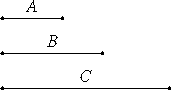# Proposition 16

If two numbers are relatively prime, then the second is not to any other number as the first is to the second.

Let the two numbers A and B be relatively prime.

I say that B is not to any other number as A is to B.

If possible as A is to B, let B be to C.Now A and B are relatively prime, numbers which are relatively prime are also least, and the least numbers measure those which have the same ratio the same number of times, the antecedent the antecedent and the consequent the consequent, therefore A measures B as antecedent antecedent.

But it also measures itself, therefore A measures A and B which are relatively prime, which is absurd.

Therefore B is not to C as A is to B.

Therefore, if two numbers are relatively prime, then the second is not to any other number as the first is to the second.

Q.E.D.

## Guide

#### Outline of the proof

Let a and b be relatively prime. Then the ratio a : b is in lowest terms. Suppose that ratio is the same as the ratio b : c. Then the antecedent of the ratio a : b, namely a, divides the antecedent of the ratio b : c, namely b. But a cannot divide b since they’re relatively prime.

#### Use of this proposition

This proposition is used in IX.18.